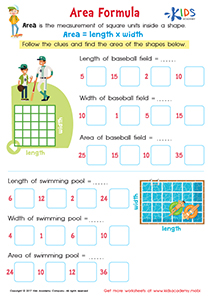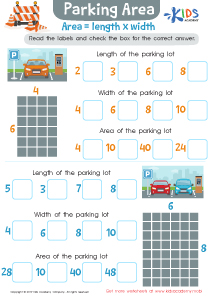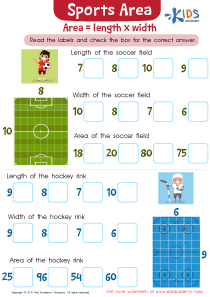# Math Lesson - Understanding Area, Grade 3

• ### Activity 1 / Area Formula WorksheetKids learn the geometric concept of area with this fun-filled area formula worksheet PDF.
Using simple explanations and a step-by-step process, kids learn the formula to calculate the area by counting squares to find the length and width, and finally multiplying them together to get area.

• ### Activity 3 / Parking Area WorksheetMath might not be your child’s favorite subject, but this does not mean that they can’t be really good at the subject. Solving math problems can seem intimidating at first glance. You can teach your kids that math equations are really not all that difficult to solve, and all they need is constant practice. In this worksheet, your kids will need to calculate the area of the parking lot, which means the length plus the width. Help them read the labels and then check the box that has the correct answer.

• ### Activity 4 / Sports Area WorksheetCalculating the area of different shapes, objects, and places should not be too difficult for your students. Help them understand it better by showing them that the area of a place is simply the length x width. This means that the length of the place will be measured, and then multiplied by the wideness. Look at the picture in the worksheet, and help your preschoolers read the labels and check the box that has the correct answer.

?